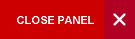Computer Applications in Physics

Semester 1 and 2

The course provides hands on training in computational techniques for physics students.  It uses a computational software package (e.g., MATLAB, SciLAB, MathCAD) and a programming language (e.g. V-Python) to aid in the analyses of data and to solve complex systems. Data processing, regression analysis, and simple programming skills will be used to simulate and model conventional physics systems. Visualization techniques will be used to display and interpret data that would have otherwise been too complex for manual analyses. Various topics in physics (e.g., projectile motion, planetary dynamics and oscillating systems) will be analyzed with emphasis on the additional complexity that computational approaches allow the scientist to handle.

Syllabus:

Introductory Material:

• Introduction to software package (e.g., MATLAB/SciLAB, MathCAD) and programming language (e.g., V-Python);
• limitations, errors and tolerances

Data organization for manipulation:

• 2-D and 3-D plots, matrices and vectors, “Least Squares” method

Functions and Equations:

• Systems of equations and approximation of functions (e.g., Taylor series, Fourier series);
• differential and state-space equations

Programming:

• Writing algorithms/programmes (e.g., Bisection method, Newton-Rhapson method);
• numerical integration

Applications:

Mandatory:

• Projectile motion with air resistance;
• Forced-Damped oscillations;
• Double-Spring oscillations;
• the wave equation, the heat equation, Poisson’s Equation.

Optional:

• Driven damped pendulum;
• Potentials and Fields;
• Navier-Stokes Equation;
• Two- and Three-body problem;
• Planetary motion;
• Fourier Analysis;
• Transients in circuits;
• Chaos;
• Molecular dynamics;
• Electrostatics;
• Diffusion;
• Phonons;
• Random systems;
• Statistical mechanics;
• Quantum mechanics.
Evaluation:

The course assessment will be conducted as follows:

Coursework

Minimum of 6 graded lab assignments of equal weighting                  10%

Course project                         20%

Two one-hour practical tests (10% each)                                  20%

Final Examination

One 2-hour final practical examination                                     50%

Students will be expected to satisfy the examiners in both components.

Learning Objectives:

At the end of the course, students should be able to:

• use computational software to organize, condition and present experimental data.
• solve systems of equations (e.g., parametric equations, multi-step radioactive decay, projectile motion) using computational software.
• simulate and model conventional physical systems (complex oscillating systems, planetary motion, multi-step radioactive decay, transient phenomena, projectile motion with air resistance, and climate evolution)
• write simple computer programmes to solve problems in physics (numerical integration, root finding by the bisection method, Taylor/Fourier series)
• apply computational visualization techniques to interpret complex data
PHYS1411 and PHYS1412 and PHYS1421 and PHYS1422

Books:

Prescribed

Giordano, N. J. and Nakanishi, H. (2005), Computational Physics, 2nd Edition; Benjamin Cummings. ISBN-10: 0131469908; ISBN-13: 978-0131469907

Highly Recommended

Landau, R. H., Paez, M. J. and Bordeianu C. C., (2007), Computational Physics: Problem Solving with Computers, 2nd Edition; Wiley-VCH. ISBN-10: 3527406263; ISBN-13: 978-3527406265

Online Resources:

http://farside.ph.utexas.edu/teaching/329/lectures/lectures.html

http://myphysicslab.com

http://physics.oregonstate.edu/~rubin/Books/eBookWorking/VideoLecs/

Course Code:
PHYS2396
Credits:
3 Credits
Level:
Level 2
Top of Page